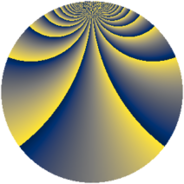# Properties

 Label 2880.2.bwLevel $2880$ Weight $2$ Character orbit 2880.bw Rep. character $\chi_{2880}(191,\cdot)$ Character field $\Q(\zeta_{6})$ Dimension $192$ Sturm bound $1152$

# Learn more about

## Defining parameters

 Level: $$N$$ $$=$$ $$2880 = 2^{6} \cdot 3^{2} \cdot 5$$ Weight: $$k$$ $$=$$ $$2$$ Character orbit: $$[\chi]$$ $$=$$ 2880.bw (of order $$6$$ and degree $$2$$) Character conductor: $$\operatorname{cond}(\chi)$$ $$=$$ $$36$$ Character field: $$\Q(\zeta_{6})$$ Sturm bound: $$1152$$

## Dimensions

The following table gives the dimensions of various subspaces of $$M_{2}(2880, [\chi])$$.

Total New Old
Modular forms 1200 192 1008
Cusp forms 1104 192 912
Eisenstein series 96 0 96

## Trace form

 $$192q + O(q^{10})$$ $$192q + 96q^{25} + 32q^{33} + 48q^{41} + 96q^{49} + 16q^{57} + 48q^{93} + O(q^{100})$$

## Decomposition of $$S_{2}^{\mathrm{new}}(2880, [\chi])$$ into newform subspaces

The newforms in this space have not yet been added to the LMFDB.

## Decomposition of $$S_{2}^{\mathrm{old}}(2880, [\chi])$$ into lower level spaces

$$S_{2}^{\mathrm{old}}(2880, [\chi]) \cong$$ $$S_{2}^{\mathrm{new}}(36, [\chi])$$$$^{\oplus 10}$$$$\oplus$$$$S_{2}^{\mathrm{new}}(144, [\chi])$$$$^{\oplus 6}$$$$\oplus$$$$S_{2}^{\mathrm{new}}(180, [\chi])$$$$^{\oplus 5}$$$$\oplus$$$$S_{2}^{\mathrm{new}}(288, [\chi])$$$$^{\oplus 4}$$$$\oplus$$$$S_{2}^{\mathrm{new}}(576, [\chi])$$$$^{\oplus 2}$$$$\oplus$$$$S_{2}^{\mathrm{new}}(720, [\chi])$$$$^{\oplus 3}$$$$\oplus$$$$S_{2}^{\mathrm{new}}(1440, [\chi])$$$$^{\oplus 2}$$